# Surface Area

## Introduction to Surface Area

Say there is a box that needs to be painted. You need to calculate the amount of paint that would be needed to cover it. Then you would need to know the sum of the areas of the six surfaces of the box (two sides, front, back, top and bottom). This total area of the six surfaces is called its surface area.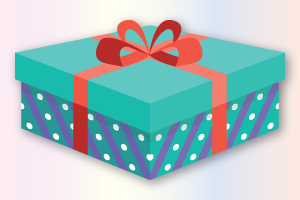## What is Surface Area?

The surface area of a three-dimensional object is the total area of all of its surfaces.

Surface area is important to know situations where we want to wrap something, paint something and eventually while building things to get the best possible design.

## How is it important?

### Surface Area of Rectangular Prism

\begin{align} {\text{Surface area of rectangular prisms}}& = {\text{sum of surface area of six faces}}\\ & = lw + lw + wh + wh + lh + lh\\ & = 2\left( {lw + wh + lh} \right) \end{align}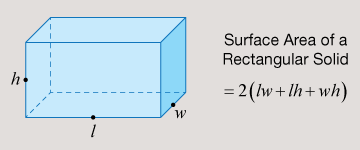### Surface Area of a Cuboid

Just like a square is a rectangle with all four sides equal. Similarly, a cuboid is a rectangular prism in which the length, breadth and height are all equal. In a cuboid we can represent the side \begin{align}a = l = b = h\end{align}. In this case

\begin{align}{\text{Surface Area of cuboid}} = 2\left( {lb + bh + lh} \right) = 2\left( {{a^2} + {a^2} + {a^2}} \right) = 6{a^2}\end{align}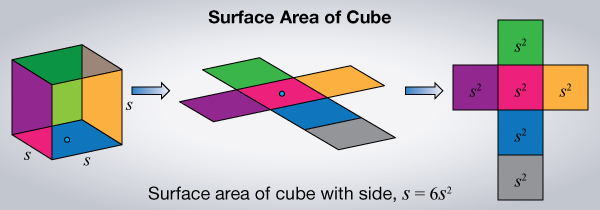A very good way to be able to visualise surface area is to “unfold” the 3-dimensional shape and lay it out flat on a surface. The area of the unfolded part is the surface area of the shape, or in this case, the cube.

### Surface Area of a Cone

We spoke about the ice cream cone some time back. The cone has a circle (say of radius $${r}$$) at its base, and a curved surface (say of height $${h}$$), and slant height $${l}$$. In this case, the surface area of the cone can be calculated thus:

\begin{align} {\text{Surface Area of Cone}}& = {\text{area of curved surface}} + {\text{area of circle}}\\ & = \pi l + \pi {r^2}\\ & = \pi r\left( {l + r} \right) \end{align}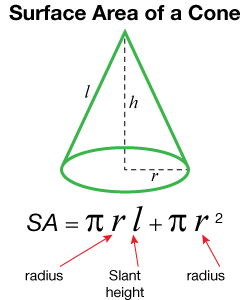Remember that the radius $${r}$$, the height $${h}$$ and the slant $${l}$$ form the three sides of a right-angled triangle and so they can be represented as $${r^2 + h^2 = l^2}$$ and this formula can be used first to calculate one of these if only the other two are given.

### Surface Area of a Cylinder

When you look at a can of soup, you are looking at a cylinder. It has a curved surface as its main body, and two circular faces at either end. Here is how you can calculate the surface area of a cylinder with radius $${r}$$ and height $${h}$$.

\begin{align} \text{Surface Area of cylinder} &= \left(\text{area of circle at top}/\text{front} + \text{area of circle at}\\ \text{bottom}/\text{back} + \text{area of curved surface} \right) \\ & = \pi {r^2} + \pi {r^2} + 2\pi rh\\ & = \pi r\left( {r + r + 2h} \right)\\ & = \pi r\text{ }\left( {2r + 2h} \right)\\ & = 2\pi r\left( {r + h} \right) \end{align}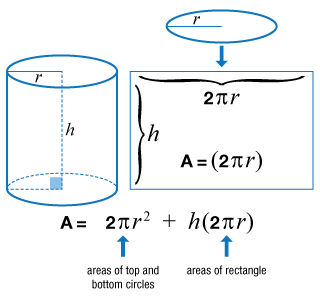## Important Topics

More Important Topics
Numbers
Algebra
Geometry
Measurement
Money
Data
Trigonometry
Calculus
More Important Topics
Numbers
Algebra
Geometry
Measurement
Money
Data
Trigonometry
Calculus
Learn from the best math teachers and top your exams

• Live one on one classroom and doubt clearing
• Practice worksheets in and after class for conceptual clarity
• Personalized curriculum to keep up with school